Anúncio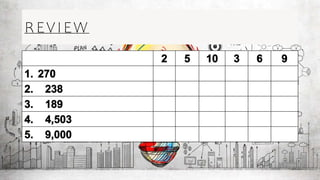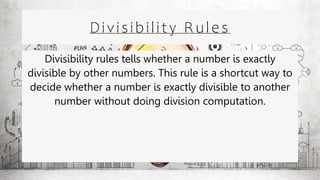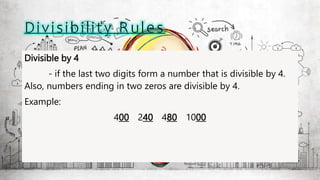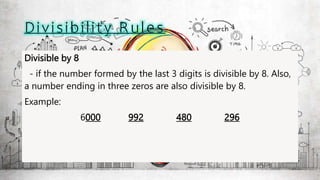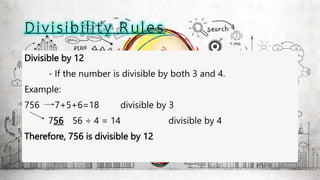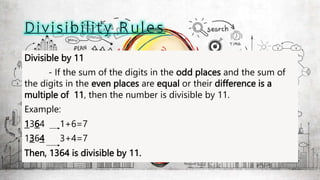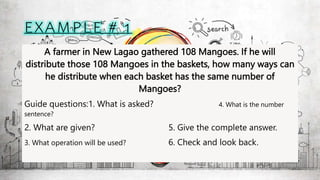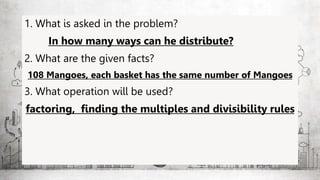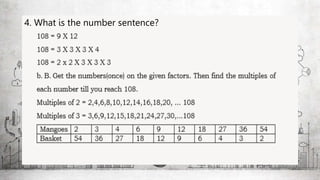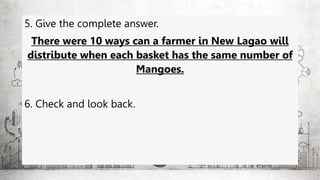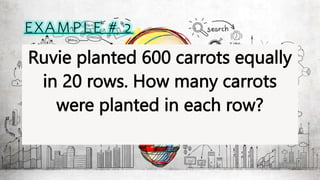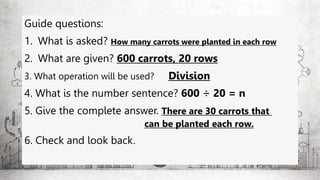1 de 17
Anúncio

### DIVISIBILITY-RULES.pptx

1. Good Morning!
2. P R E P A R E D B Y : M S . C R I S T H E L J O Y Q . M A C A J E T O
3. REVIEW
4. Factors - a number or algebraic expression that divides another number or expression evenly. Multiples - is the result of multiplying a number by an integer Factor? Multiple?
5. Divisibility Rules Divisibility rules tells whether a number is exactly divisible by other numbers. This rule is a shortcut way to decide whether a number is exactly divisible to another number without doing division computation.
6. Divisible by 4 - if the last two digits form a number that is divisible by 4. Also, numbers ending in two zeros are divisible by 4. Example: 400 240 480 1000
7. Divisible by 8 - if the number formed by the last 3 digits is divisible by 8. Also, a number ending in three zeros are also divisible by 8. Example: 6000 992 480 296
8. Divisible by 12 - If the number is divisible by both 3 and 4. Example: 756 7+5+6=18 divisible by 3 756 56 ÷ 4 = 14 divisible by 4 Therefore, 756 is divisible by 12
9. Divisible by 11 - If the sum of the digits in the odd places and the sum of the digits in the even places are equal or their difference is a multiple of 11, then the number is divisible by 11. Example: 1364 1+6=7 1364 3+4=7 Then, 1364 is divisible by 11.
10. A farmer in New Lagao gathered 108 Mangoes. If he will distribute those 108 Mangoes in the baskets, how many ways can he distribute when each basket has the same number of Mangoes? Guide questions:1. What is asked? 4. What is the number sentence? 2. What are given? 5. Give the complete answer. 3. What operation will be used? 6. Check and look back.
11. 1. What is asked in the problem? In how many ways can he distribute? 2. What are the given facts? 108 Mangoes, each basket has the same number of Mangoes 3. What operation will be used? factoring, finding the multiples and divisibility rules
12. 4. What is the number sentence?
13. 5. Give the complete answer. There were 10 ways can a farmer in New Lagao will distribute when each basket has the same number of Mangoes. 6. Check and look back.
14. Ruvie planted 600 carrots equally in 20 rows. How many carrots were planted in each row?
15. Guide questions: 1. What is asked? How many carrots were planted in each row 2. What are given? 600 carrots, 20 rows 3. What operation will be used? Division 4. What is the number sentence? 600 ÷ 20 = n 5. Give the complete answer. There are 30 carrots that can be planted each row. 6. Check and look back.
Anúncio